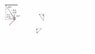# Tangential and Radial Acceleration problem

• phoenix20_06

#### phoenix20_06

Hello,
This is my problem and the solution from the book. I don't understand how they came to the solution. I believe it's wrong and I offer my own solution(but incomplete). I would appreciate if you could explain how they arrived to their solution or if I'm right. Thanks:)

## Homework Statement

A ball swings in a vertical circle at the end of a rope 1.50 m
long. When the ball is 36.9° past the lowest point on its
way up, its total acceleration is (-22.5 i + 20.2j) m/s^2. At
that instant, (a) sketch a vector diagram showing the components
of its acceleration, (b) determine the magnitude
of its radial acceleration, and (c) determine the speed and
velocity of the ball.

2. Solution from the book

http://photos-a.ak.fbcdn.net/hphotos-ak-snc1/hs030.snc1/3190_91017112564_509877564_2512520_5779508_n.jpg [Broken]

3. My Confusion :-)

First of all, the angle seems to be in a wrong place. A friend suggested that they ran out of space and put it below x-axis instead of under the ).

Second, I don't understand their reason for breaking total acceleration into components and using them in calculation of Ac.

## The Attempt at a Solution

TotalA = A.radial(Ar) + A.tangential (At) Because all 3 are vectors, then either the i or j is Ac. I believe this is uniform circular motion where v stays the same all the time, so At = 0. then Ac = totalA ?

However, the book gives a different answer, and I cannot imagine how they came up with this odd relationship between 2 vectors.

P.S. this is not my home work (it's just a problem I found in my book while preparing for a final exam.), but I'm worried something like that will be on my final.

R.M.

Last edited by a moderator:
I believe this is uniform circular motion where v stays the same all the time, so At = 0. then Ac = totalA ?
It is true only in the horizontal circle, not in the vertical circle. The given problem is a motion in vertical circle.

Thank you for the reply. Unfortunately, it doesn't change anything in my progress :-(

I found out that the angle between Atot and AC is 11.2 degrees and from there I don't see a solution

Last edited:
What confuses me is the calculation of AC in the solutions...

Ac = (22.5)cos(53.1) + (20.2)cos36.9 = 29.7

It seems that (22.5)cos(53.1) implies that 22.5 is a hypotenuse in some triangle that got formed using Atot components. The same for 20.2. I don't see how ...:( Even if this gets us Ac's x and y...shouldn't we use pyth. theorem to find Ac's magnitude?

Ac = sqrt (ACx^2 +ACy^2) ?

Second, I don't understand their reason for breaking total acceleration into components and using them in calculation of Ac.
At the given position of the ball, they have given the components of the total acceleration which includes radial and tangential acceleration. The have taken the components of the components of A(tot) along the rope to find the Ac.
Ac = (22.5)cos(53.1) + (20.2)cos36.9 = 29.7
22.5 makes an angle 53.1 with the rope and 20,2 makes an angle 36.9 with the rope.

Last edited:
I understand that the coordinates include Radial...I'm not sure what transverse acceleration is...(is it tangent acceleration?)
What I don't understand is the geometry that has to be used in with only 2 vectors.
If I had tangential acceleration, finding radial would be piece of cake...

I know that Magnitude of Ac should be greater than magnitude of Ar. So I tried to graph both vectors.
I know that the angle between the ball and Ac is 53.1* and between Atot and the Ball is 41.9*. Assuming I'm right, this tells me that the y component of Ac is 22.02.
Then AC should be 22.02 /cos36.9 = 25.2 m/s^2 ?
I know it's hard to explain without a graph, but please bare with me:)

attaching a file with a picture that may clear the things up.
good luck

#### Attachments

•Untitled.jpg
5.8 KB · Views: 833
What confuses me is the calculation of AC in the solutions...

Ac = (22.5)cos(53.1) + (20.2)cos36.9 = 29.7
You are given the acceleration in terms of its horizontal and vertical components. What you need is the radial acceleration, so take the radial components of the given components.

It seems that (22.5)cos(53.1) implies that 22.5 is a hypotenuse in some triangle that got formed using Atot components. The same for 20.2. I don't see how
That's true. For example, the given horizontal component is a vector. You want its component in the radial direction, so it becomes the hypotenuse of a right triangle. One side will be parallel to the radius, the other will be perpendicular to it.

...:( Even if this gets us Ac's x and y...shouldn't we use pyth. theorem to find Ac's magnitude?

Ac = sqrt (ACx^2 +ACy^2) ?
No. You'd use Pythagorus to find the resultant of two perpendicular components. But here you are just finding the radial component of each vector and adding them up--they are in the same direction, not perpendicular.

Thank you very much all, I think I understand now